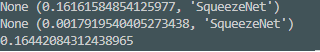# Why is torch.jit.script slower?

I compared the performance(speed) of Torchvision’s Squeezenet original model with `torch.jit.script(model)` which I expected to speed up because Torchscript was asynchronous, but the original model was faster. What’s the reason? Which one did I miss?scripted model : 0.2353s
original model : 0.1644s

it is not asynchronous (beyond cuda kernel launches, which is not related to jit), just python-less execution mode with optimizations

one thing I’ve seen, is that some jitted operations incorrectly enable requires_grad

but simpler explanation is that you’re not measuring it right - time the THIRD call of compiled model (actually, from your screenshot it seems you’re compiling twice [i.e. two model objects], which is also incorrect). reason is that profiling mode executor creates optimized bytecode on second call.

Thanks for replying.
The time was measured separately between `torch.jit.script` and the original model.
like this,

``````torch.jit.script(models.squeezenet1_0(pretrained=True).cuda().eval())
torch.jit.script(models.squeezenet1_0(pretrained=True).cuda().eval())
#models.squeezenet1_0(pretrained=True).cuda().eval()
#models.squeezenet1_0(pretrained=True).cuda().eval()
``````

Then result is``````#torch.jit.script(models.squeezenet1_0(pretrained=True).cuda().eval())
#torch.jit.script(models.squeezenet1_0(pretrained=True).cuda().eval())
models.squeezenet1_0(pretrained=True).cuda().eval()
models.squeezenet1_0(pretrained=True).cuda().eval()
``````Why exactly is Torchscript slower than pytorch?

as I said, do something like:
net = jit.script(models.squeezenet1_0(pretrained=True).cuda().eval())
net(x); net(x)

and only then measure time (e.g. %timeit net(x))

As you say, Torchscript is faster.
Is this the result of different ways of optimizing it?
I want a detailed explanation.

In a nutshell,

1. “compilation” analyzes whole functions, with knowledge about variable types - some optimizations are done at this level (e.g. dead code elimination)
2. python bytecode interpreter is not used to execute generated code - more specialized executor for statically typed code supposedly works faster
3. fusion optimizations further compile specialized cuda kernels, so e.g. a.mul(b).add(c) is computed in one go
4. some patterns have specialized optimizations, e.g. conv+batchnorm